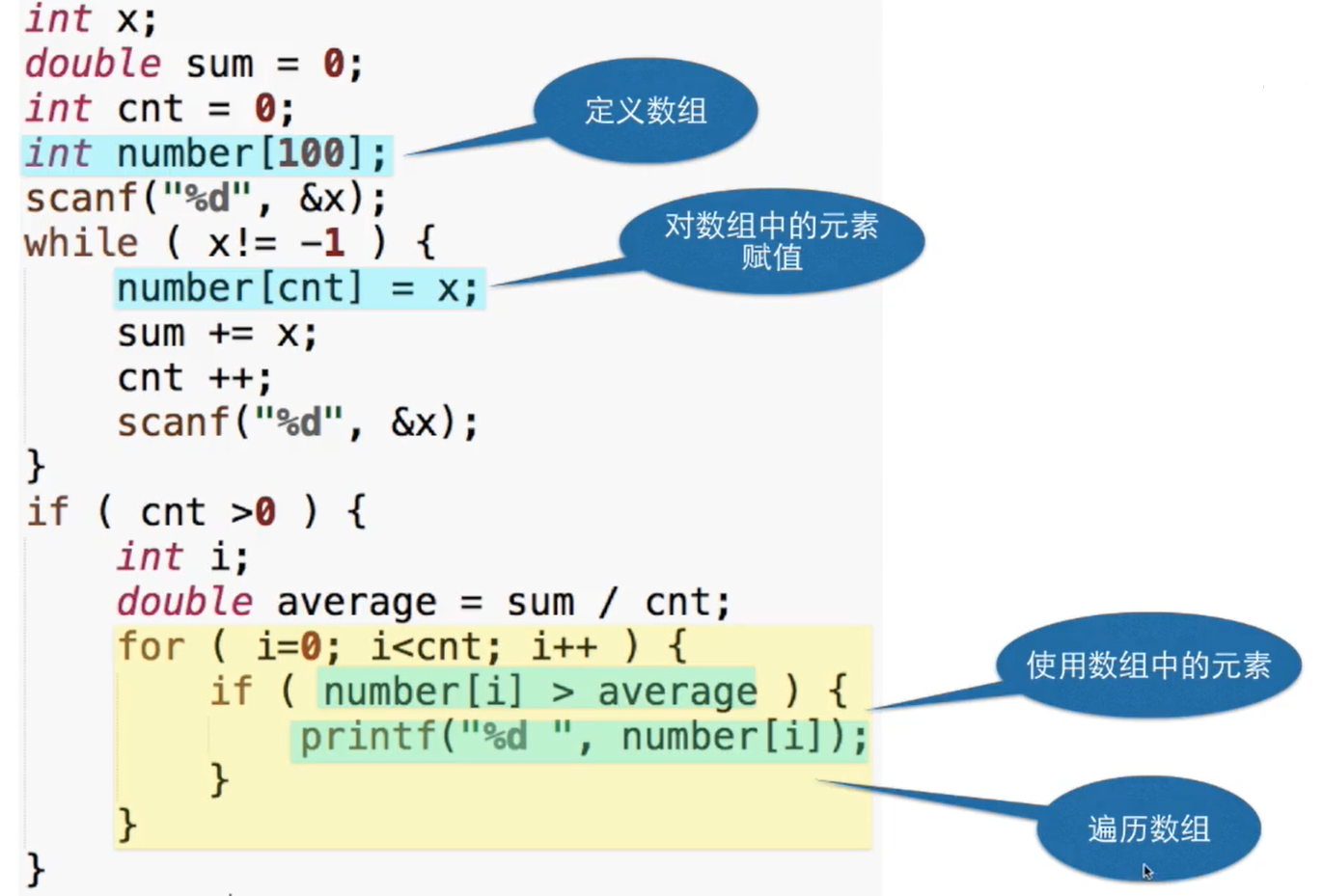``````int x;
scanf("%d",&x);
int sum = 0;
int cnt = 0;
while(x > -1){
sum += x;
cnt++;
scanf("%d",&x);
}
if(cnt > 0){
printf("平均数为%f",sum * 1.0 / cnt);
}``````

::: tip 上述程序判断cnt>0目的是保证确有数字输入。 上述程序不需要记录输入的每一个数字。 :::

``````#include <stdio.h>

int main()
{
int x;
scanf("%d",&x);
int sum = 0;
int cnt = 0;
int number;
while(x > -1){
number[cnt] = x;
sum += x;
cnt++;
scanf("%d",&x);
}
if(cnt > 0){
printf("平均数为%f\n",sum * 1.0 / cnt);
}
int i = 0;
for(i;i < cnt;i++){
if (number[i] > sum * 1.0 / cnt)
printf("%d ",number[i]);
}
return 0;
}``````
``````5
4
3
2
1
0
-1

5 4 3
--------------------------------
Process exited after 3.95 seconds with return value 0``````::: warning 上述程序存在安全隐患，原因是没有判断输入数字的数量是否会超出数组number中规定的最大数量100。 :::Suzhou
Lv1

17

9

0